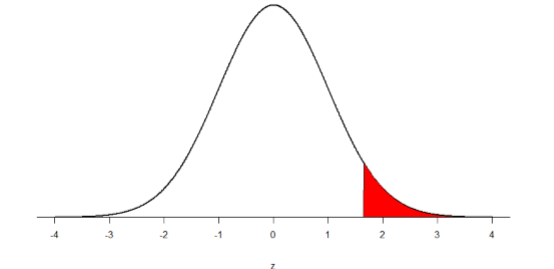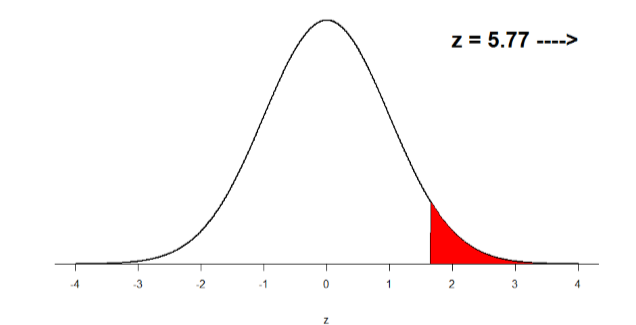# 7.9: Office Temperature

$$\newcommand{\vecs}{\overset { \rightharpoonup} {\mathbf{#1}} }$$ $$\newcommand{\vecd}{\overset{-\!-\!\rightharpoonup}{\vphantom{a}\smash {#1}}}$$$$\newcommand{\id}{\mathrm{id}}$$ $$\newcommand{\Span}{\mathrm{span}}$$ $$\newcommand{\kernel}{\mathrm{null}\,}$$ $$\newcommand{\range}{\mathrm{range}\,}$$ $$\newcommand{\RealPart}{\mathrm{Re}}$$ $$\newcommand{\ImaginaryPart}{\mathrm{Im}}$$ $$\newcommand{\Argument}{\mathrm{Arg}}$$ $$\newcommand{\norm}{\| #1 \|}$$ $$\newcommand{\inner}{\langle #1, #2 \rangle}$$ $$\newcommand{\Span}{\mathrm{span}}$$ $$\newcommand{\id}{\mathrm{id}}$$ $$\newcommand{\Span}{\mathrm{span}}$$ $$\newcommand{\kernel}{\mathrm{null}\,}$$ $$\newcommand{\range}{\mathrm{range}\,}$$ $$\newcommand{\RealPart}{\mathrm{Re}}$$ $$\newcommand{\ImaginaryPart}{\mathrm{Im}}$$ $$\newcommand{\Argument}{\mathrm{Arg}}$$ $$\newcommand{\norm}{\| #1 \|}$$ $$\newcommand{\inner}{\langle #1, #2 \rangle}$$ $$\newcommand{\Span}{\mathrm{span}}$$

Let’s do another example to solidify our understanding. Let’s say that the office building you work in is supposed to be kept at 74 degree Fahrenheit but is allowed to vary by 1 degree in either direction. You suspect that, as a cost saving measure, the temperature was secretly set higher. You set up a formal way to test your hypothesis.

Step 1: State the Hypotheses You start by laying out the null hypothesis:

$$H_0$$: There is no difference in the average building temperature

$$H_0: \mu = 74$$

Next you state the alternative hypothesis. You have reason to suspect a specific direction of change, so you make a one-tailed test:

$$H_A$$: The average building temperature is higher than claimed

$$\mathrm{H}_{\mathrm{A}}: \mu>74$$

Step 2: Find the Critical Values You know that the most common level of significance is $$α$$ = 0.05, so you keep that the same and know that the critical value for a one-tailed $$z$$-test is $$z*$$ = 1.645. To keep track of the directionality of the test and rejection region, you draw out your distribution:Figure $$\PageIndex{1}$$: Rejection region

Step 3: Calculate the Test Statistic Now that you have everything set up, you spend one week collecting temperature data:

Table $$\PageIndex{1}$$: Temperature data for a week
Day Temperature
Monday 77
Tuesday 76
Wednesday 74
Thursday 78
Friday 78

You calculate the average of these scores to be $$\overline{\mathrm{X}}$$= 76.6 degrees. You use this to calculate the test statistic, using $$μ$$ = 74 (the supposed average temperature), $$σ$$ = 1.00 (how much the temperature should vary), and $$n$$ = 5 (how many data points you collected):

$z=\dfrac{76.60-74.00}{1.00 / \sqrt{5}}=\dfrac{2.60}{0.45}=5.78 \nonumber$

This value falls so far into the tail that it cannot even be plotted on the distribution!Figure $$\PageIndex{2}$$: Obtained $$z$$-statistic

Step 4: Make the Decision You compare your obtained $$z$$-statistic, $$z$$ = 5.77, to the critical value, $$z*$$ = 1.645, and find that $$z > z*$$. Therefore you reject the null hypothesis, concluding:

Based on 5 observations, the average temperature ($$\overline{\mathrm{X}}$$= 76.6 degrees) is statistically significantly higher than it is supposed to be, $$z$$ = 5.77, $$p$$ < .05.

Because the result is significant, you also calculate an effect size:

$d=\dfrac{76.60-74.00}{1.00}=\dfrac{2.60}{1.00}=2.60 \nonumber$

The effect size you calculate is definitely large, meaning someone has some explaining to do!

7.9: Office Temperature is shared under a CC BY-NC-SA 4.0 license and was authored, remixed, and/or curated by Foster et al. (University of Missouri’s Affordable and Open Access Educational Resources Initiative) via source content that was edited to conform to the style and standards of the LibreTexts platform; a detailed edit history is available upon request.